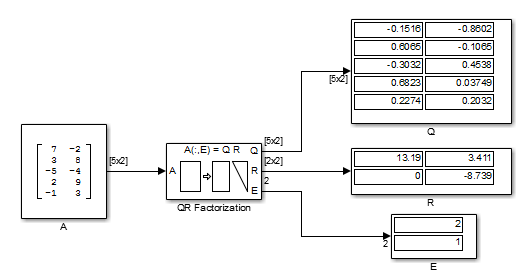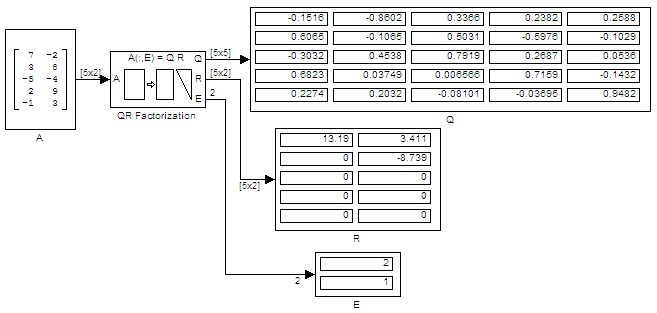# Factor Arbitrary Matrix Using QR Factorization Block

The Output size parameter of the QR factorization block has two settings: `Economy` and `Full`. When the M-by-N input matrix A has dimensions such that M > N, the dimensions of output matrices Q and R differ depending on the setting of the Output size parameter. However, if the size of the input matrix A is such that M is less than or equal to N, output matrices Q and R have the same dimensions, regardless of whether the Output size is set to `Economy` or `Full`.

The input to the QR Factorization block in the following model is a 5-by-2 matrix A. When you change the setting of the Output size parameter from `Economy` to `Full`, the dimensions of the output given by the QR Factorization block also change.

Open the `ex_qrfactorization_ref` model.Double-click the QR Factorization block, set the Output size parameter to `Economy`, and run the model.The QR Factorization block outputs a 5-by-2 matrix Q and a 2-by-2 matrix R.

Change the Output size parameter of the QR Factorization block to `Full` and rerun the model. The QR Factorization block outputs a 5-by-5 matrix Q and a 5-by-2 matrix R.